# 第二章：谐波¶

## 2.1 三角波¶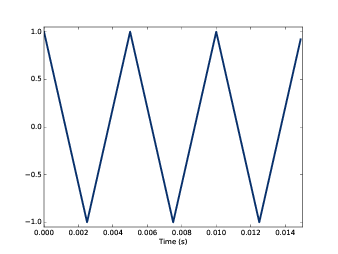class TriangleSignal(Sinusoid):

def evaluate(self, ts):
cycles = self.freq * ts + self.offset / PI2
frac, _ = np.modf(cycles)
ys = np.abs(frac - 0.5)
ys = normalize(unbias(ys), self.amp)
return ys


TriangleSignal 继承自 Sinusoid ， 因此它同样包含 freqampoffset 三个属性。 不同在于它复写了 evaluate 。其中 ts 依然是采样点的时间，我们来看看这个 evaluate 是如何产生 三角波的：

1. cycles 表示从采样点的循环数。 np.modf 把它的小数部分提取出来放到 frac 中，它的整数部分 被丢弃了 
2. frac 现在是一个给定频率的0~1变化的斜坡信号，将它减去0.5会使其范围变到-0.5~0.5。然后取绝对值后， 它的值就成了从0增加到0.5，再从0.5减小到0
3. unbias 会把整个信号向下移动，使其相对于y轴居中，然后 normalize 将信号的幅度放大到 amp

signal = thinkdsp.TriangleSignal(200)
signal.plot()


wave = signal.make_wave(duration=0.5, framerate=10000)
spectrum = wave.make_spectrum()
spectrum.plot()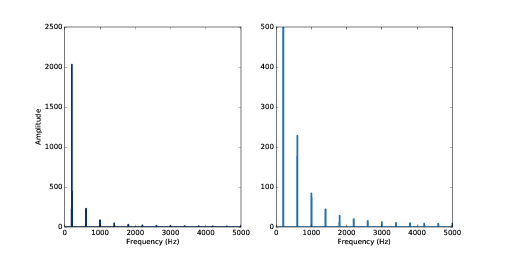## 2.2 方波¶

thinkdsp 还提供了 SquareSignal 类来表示方波信号，这个类的定义如下:

def evaluate(self, ts):
cycles = self.freq * ts + self.offset / PI2
frac, _ = np.modf(cycles)
ys = self.amp * np.sign(unbias(frac))
return ys


SquareSignalevaluate 方法也具有类似的结构。其中 ts 依然是采样时间序列， frac 是它的小数部分，它的值从0到1周期的变化。

unbiasfrac 调整到-0.5~0.5，然后 np.sign 将结果的负值映射到-1，正值映射到1。 最后，乘以 amp 将信号的幅值调整到 -amp~amp 。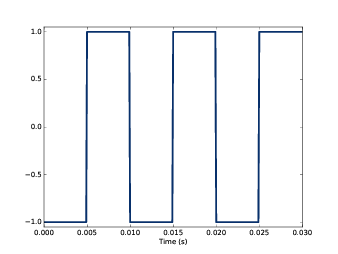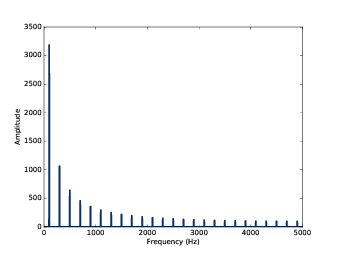## 2.3 混叠¶framerate = 10000

signal = thinkdsp.CosSignal(4500)
duration = signal.period*5
segment = signal.make_wave(duration, framerate=framerate)
segment.plot()

signal = thinkdsp.CosSignal(5500)
segment = signal.make_wave(duration, framerate=framerate)
segment.plot()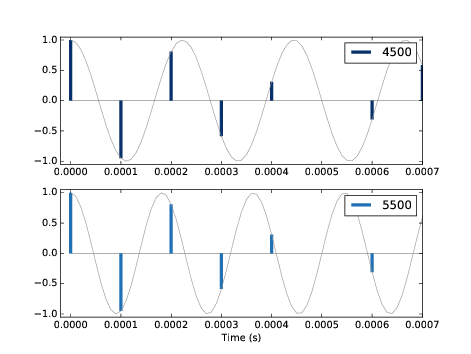## 2.4 频谱的计算¶

from np.fft import rfft, rfftfreq

# class Wave:
def make_spectrum(self):
n = len(self.ys)
d = 1 / self.framerate

hs = rfft(self.ys)
fs = rfftfreq(n, d)

return Spectrum(hs, fs, self.framerate)


self 参数代表的是波形对象本身， n 是波形的采样点数目， d 是采样率的倒数，也就是采样时间步长。

np.fft 是Numpy提供的FFT方法（一种高效的计算DFT的算法）。

make_spectrum 使用了 rfft ，它的意思是“实数FFT”，如果信号是实数而不是复数，我们就可以使用它。 之后，我们会看到“完整FFT”，它可以处理复信号（见 7.9 ）。 rfft 的结果 hs 是一个复数的Numpy数组， 它表示了各个频率分量的复数幅值（幅度和初始相位另一种表示形式）。

rfftfreq 的结果 fs 包含了与 hs 对应的频率值。

• 复数是实部和虚部的和，通常写成： $$x + iy$$ ，其中 $$i$$ 是单位虚数， 也就是 $$\sqrt { - 1}$$ 。 我们可以把复数的x和y看做是复数在复平面下的坐标（以实轴为横坐标，虚轴为纵坐标的直角坐标系）
• 复数也可以表示为幅值和复指数的形式，写成： $$A{e^{i\varphi }}$$ ，其中 $$A$$ 为模， $$\varphi$$ 为幅角。我们可以把它看做是复数在极坐标下的表示。

$$x + iy$$ 的极坐标表示为： $$A\cos (\varphi ) + A\sin (\varphi )i$$ ， 根据欧拉公式 $${e^{ix}} = \cos (x) + isin(x)$$ ，可以得出 $$A{e^{i\varphi }}$$

hs 中的每个复数就代表了该频率分量的复数幅值：它的模值就是该频率的幅度，它的幅角就是该频率的初始相位。

Spectrum 类中提供了两个只读的属性： ampsangles ， 用来得到这些幅值和初始相位（它们都被放在Numpy数组中）。 我们在画频谱图的时候，一般会画出相对于 fs 下的 amps ，或者相对于 fsangles 。 在实际使用中，我们几乎不会直接去关注 hs 的实部和虚部。

spectrum.hs *= 2
spectrum.hs[spectrum.fs > cutoff] = 0


Spectrum 类中提供了简单的方法来完成这两个操作:

spectrum.scale(2)
spectrum.low_pass(cutoff)


## 2.5 练习¶

1. 生成一个440Hz的三角信号，生成并画出它0.01s的波形。
2. 生成频谱并打印出 hs ，看看他的幅值和初始相位是多少？
3. 设置 hs=100 ，看看这样做会在波形上产生什么样的影响。 提示：频谱对象有一个 make_wave 方法可以生成对应的波形。

1. 画出原始的频谱图
2. 调用你写的函数，并画出计算得到的频谱图
3. 使用 make_wave 生成这个频谱的波形，并听听看产生了什么效果。

  使用下划线代表一个变量的时候，表示之后不会使用它，这是一个编码惯例。